## Firebird VIN Decoding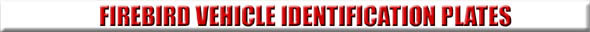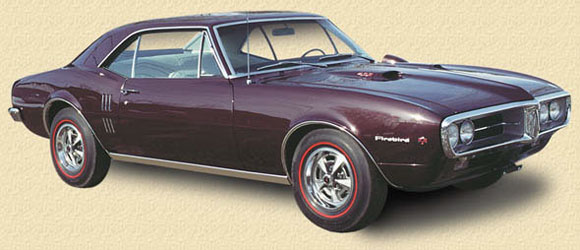1967 models have the Vehicle Identification Number located on a stainless-steel plate riveted to the left door jamb on the hinge post facing. 1968-71 models have a plate visible through the windshield on the driver’s side dash panel.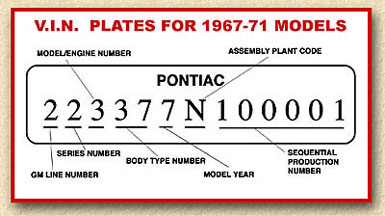6th digit: Last digit of the model year: 7=1967 8=1968 9=1969 0=1970 1=1971 7th digit: Letter indicating assembly plant: L=Van Nuys, California N=Norwood, Ohio U=Lordstown, Ohio  8th through 12th digits:Sequential production number starting with 600001 for 6-cylinder models and 100001 for V8 models.
1st digit: GM line number:
Pontiac=2

2nd digit: Series number:
Firebird=2

3rd digit: Model/Engine number:
3=All 1967-69 models
3=Standard models(1970-71)
4=Esprit V8(1970-71)
6=Formula V8 (1970-71)
8=Trans Am V8 (1970-71)

4th and 5th digit: Body type number:
37=Sport Coupe (1967-69)
67=Convertible (1967-69)
87=Sport Coupe (all 1970-81)

 This V.I.N. plate is visible through the windshield on the driver’s side dash panel.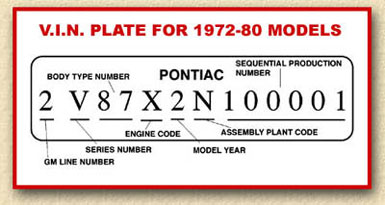1st digit: GM line number: Pontiac=2  2nd digit: Series number: S=Base Firebird T=Esprits U=Formula V=Trans Am (1972-74) W=Trans Am (1975-1980) X=10th Anniversary Trans Am (1979) X=Turbo Indy Trans Am (1980) 3rd and 4th digit: Body type number: 87=Sport Coupe 5th digit: Engine code: A=231 c.i.d. 2-bbl V6 (1978-80) C=231 c.i.d. 2-bbl V6 (1977) D=250 c.i.d. 1-bbl L6 (1972-76) G=305 c.i.d. 2-bbl V8 (1979) H=305 c.i.d. 4-bbl V8 (1975) H=305 c.i.d. 4-bbl V8 (1980) J=350 c.i.d. 4-bbl V8 (1976) K=403 c.i.d. 4-bbl V8 (1977-79) L=350 c.i.d. 4-bbl V8 (1978) M=350 c.i.d. 2-bbl V8, single exh. (1972-76) N=350 c.i.d. 2-bbl V8, dual exh. (1972-74) R=400 c.i.d. 2-bbl V8, single exh. (1972-74) P=400 c.i.d. 2-bbl V8, dual exh. (1973-74) P=350 c.i.d. 4-bbl V8 (1977) R=350 c.i.d. 4-bbl V8 (1977) S=400 c.i.d. 4-bbl V8 (1975-76) S=265 c.i.d. 2-bbl V8 (1980) T=400 c.i.d. 4-bbl V8, dual exh. (1972-74) T=301 c.i.d. TC 4-bbl V8 (Turbo) (1980) U=305 c.i.d. 2-bbl V8 (1978)
W=455 c.i.d. 4-bbl V8 (1975-76)
W=301 c.i.d. 4-bbl V8 (1979-80)
X=455 c.i.d. 300 hp 4-bbl V8 H.O. (1972)
X=455 c.i.d. 4-bbl V8 S.D. (1973-74)
Y=455 c.i.d. 4-bbl V8 (1973-74)
Y=301 c.i.d. 2-bbl V8 (1977, 1979)
Y=301 c.i.d. 4-bbl V8 T/A 4.9 (1980)
Z=400 c.i.d. 4-bbl V8 (1977-79)

6th digit: Last digit of the model year:
2=1972
3=1973
4=1974
5=1975
6=1976
7=1977
8=1978
9=1979
A=1980

7th digit: Assembly plant location:
L=Van Nuys, California
N=Norwood, Ohio

8th through 13th digits:Sequential
production number starting with 500001
for all 1972 models and 100001 for all
1973-80 models.

 The V.I.N. plate for this year is visible through the windshield on the driver’s side dash panel.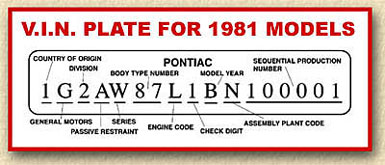1st digit: Country of Origin: 1=U.S.A. 2=Canada 2nd digit: G=General Motors  3rd digit: Division: 2=Pontiac 4th digit: Restaint system: A=Non-passive B=Automatic belts C=Inflatable restraint  5th digit: Series: S=Base Firebird T=Esprit V=Formula W=Trans Am X=Trans Am Special Edition Y=NASCAR Turbo Trans Am Pace Car
6th and 7th digits: Body style:
87=Sport Coupe

8th digit: Engine:
A=231 c.i.d. 110 hp 2-bbl V6
H=305 c.i.d. 145 hp 4-bbl V8
S=265 c.i.d. 120 hp 2-bbl V8
T=301 c.i.d. 200 hp TC 4-bbl V8 (Turbo)
W=301 c.i.d. 135 hp 4-bbl V8
W=301 c.i.d. 150 hp T/A 4.9 V8
L=350 c.i.d. 175 hp 4-bbl V8

9th digit: Check digit

10th digit: Year:
B=1981

11th digit: Assembly plant:
L=Van Nuys, California
N=Norwood, Ohio

12th through 17th digits:Sequential
production number starting with 100001
for all models.

## Subscribe to our mailing list

* indicates required